Campus St. Jérôme Av. Escadrille Normandie-Niemen 13397 Marseille Cedex 20 - France# Delocalization, Mesomerism and Simple Hückel Theory: bases of a free Java Applet

Nicolas Goudard, Yannick Carissan, Denis Hagebaum-Reignier et Stéphane Humbel

Institut des Sciences Moléculaires de Marseille UMR 7313 CNRS
Aix-Marseille UniversitéHomedownloadHuLiS

# Introduction

1. Delocalization between atoms OM-CLAO and Simple Hückel Φ=caχa + cbχbd,Ed)
2. Delocalization between states : Mesomerism Ψm=cIΨI + cIIΨII, (Ψm,Em)

# HuLiS, a Java applet1. The Hückel-Lewis CI approach
1. The scheme
2. Weights
2. The Hückel-Lewis Projected approach
1. The scheme
2. Overlap between determinants
3. Student's work in Organic Chemistry course
1. A first look at the interface
2. Example : the acrolein molecule
• Simple Hückel calculations
• Hückel CI calculations

# Abstract

Hückel-Lewis is a new scheme, designed to obtain the weights of Lewis contributing structures from adapted Hückel calculations. It has been implemented as “HuLiS”, a free Java 1.5 applet, available from http://www.hulis.free.fr. The recent html5 version is available from http://m.hulis.free.fr/hulis.html for smartphones. HuLiS is based on two of our recent publications on the subject of mesomerism [1,2]. The basics of the Hückel-Lewis approach, and of electronic delocalization in general, are shown here as a parallel between atomic orbital interaction [Φ=caχa + cbχb ] and contributing structures interaction[Ψ=cIΨI + cIIΨII ]. The results obtained with the Hückel-Lewis method compare with that of an ab initio approach.

# Introduction

Electronic delocalization has mainly two aspects: Bonds and Conjugation. The chemical bond is an electron pair sharing between two atoms. It is an electronic delocalization between two atoms. Conjugation is an electronic (π) delocalization between bonds. Both concepts are taught to students along chemistry courses.

We shall here make a parallel between the two types of electronic delocalizations. We present and apply a modified version of the numerical approach of the first (atomic) delocalization (Simple Hückel theory) to the second (Lewis). The fundamentals of this new Hückel-Lewis approach has been published recently by our group. We shall remind here these fundamentals on the simple case of a two-structure mesomerism.

Huckel theory gives access to delocalization energy. It is shown here that weights of the Lewis structures can be computed within the Huckel model. A parallel is done here between charges and weights.

## Delocalization between two atoms OM-CLAO and Hückel [Φ=capa + cbpb (Ψd,Ed) ]

The electronic delocalization between two atoms is taught within the simple qualitative approach of the Molecular Orbitals by Linear Combinaison of Atomic Orbitals (MO-LCAO). Scheme 1a shows how two overlapping atomic orbitals interact and generate π bonding / π* anti-bonding orbitals. Those can be populated with an appropriate number of electrons, so bond order and other charge delocalization quantities can be qualitatively discussed, even for unsymmetrical systems.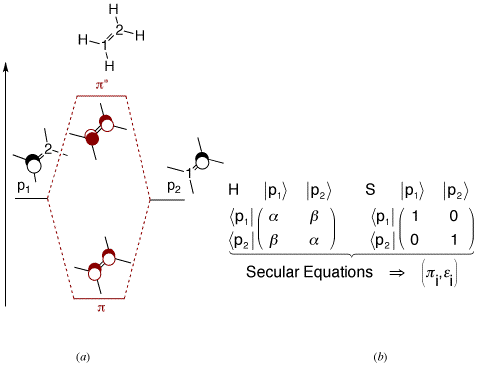Scheme 1 : (a) Orbital qualitative interaction : π and π* orbitals. (b) Quantum empirical resolution (Simple Hückel): α and β are negative energetic quantities. The molecular orbitals π and π* have an energy of α+ β and α- β, respectively.

Complementary to this qualitative approach, the well-known Simple Hückel quantum empirical method is widely taught for a better understanding of bonding in π delocalized systems, between two or several AO's (Scheme 1b). The scheme works well for π systems because the interaction is fairly constant there, so it can be parameterized quite easily. Moreover, the physical/mathematical technique used there can be exported to any two interacting systems.

Before doing so, we shall first remind that the diagonal terms of the Hamiltonian matrix ( α ) can be read as the energy of the isolated AO's (those are Carbon p electrons for instance). Similarly, the off-diagonal terms ( β ) represents the interaction between the AO's. Setting β to zero would isolate one AO's from the other. It would have numerically cut the π bond. However, σ electrons cannot be affected there because Simple Hückel theory lays on an initial definition of the σ skeleton.

Second, we shall remind that Simple Hückel theory reports all the interactions to the Hamiltonian matrix, and leaves the S overlap matrix as the identity matrix. This subtle interplay between the two matrices justifies the use of orthogonal AO's throughout the Hückel scheme. This convenient approximation is done, and works well, because we implicitly report to the H matrix all the overlap tasks, via the (negative) β parameter (Scheme 2).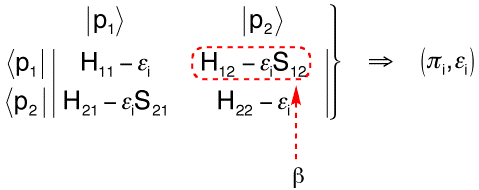Scheme 2 : Secular determinant in Simple Hückel Theory, the off diagonal terms in simple Hückel Theory.

Yet the Simple Hückel scheme gives many information for reactivity, as well as quite a few structural interesting data such as bond indices, partial atomic charges, etc, all those data come from the electronic delocalization that occurs throughout the molecule. They are taught at the undergrad level or so.

## Delocalization between states :Mesomerism. Ψm=cIΨI + cIIΨII, (Ψm,Em)

Mesomerism (or Resonance) is also taught (1st year). At this time it is essentially a graphical concept with appropriate arrows that delocalized the bonds at different locations of a molecule. We can get some hint about this electronic delocalization with this simple and efficient graphical tool. In usual π systems, such as the formamide for instance, delocalization is shown by the resonance between "contributing Lewis structures" (scheme 3). Those can be called localized configuration in the sense that the electrons are localized at some parts of the molecule. They can be localized either on only one atom (lone pairs) or localized between only two atoms (bonding pairs). Numerically the localization is done by setting to zero the appropriate off-diagonal terms of the Hamiltonian matrix that describes the delocalized system.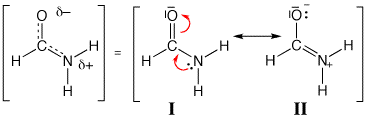Scheme 3: Resonance in formamide

Some contributing structures may have some relevance to describe a chemical system: they actually contribute to delocalize the electrons of the system. Some are not important (they don't contribute much). We usually say that a structure without charge separation can dominate in a mesomeric description of a system. However, we do not quantitatively describe the mesomerism, neither in terms of weight of a contribution (as percentages), nor in terms of stabilization energies. We rather teach rules for sorting the contributing Lewis structures by order of importance, say by weights.

Expressed in a way that reminds Asimov's Three Laws of Robotics, they can be summarized as:

• Rule #1 : In any case, the more the octet rule is fulfilled, the better .
• Rule #2 : The less charge separation, the better, except where it would conflict with rule #1.
• Rule #3 : The best structure is consistent with the electronegativity of the atoms (e.g. a negative charge is best on the most electronegative atom), except where it would conflict with rule #1 or #2.
Following the rules, one can unambiguously claim that I in formamide is more important, say it weights more, than II, because of the Second Rule.

There did not exist any simple numerical method to know how much weight a given structure among others. We built an approach that answers the question and we propose a free Java applet.

# HuLiS, a Java applet

The Java applet is called HuLiS, as an acronym for Huckel LewiS. It is freeware, and can be launch from http://www.hulis.free.fr (Figure 1). The user must have installed a Java Virtual Machine (JVM) on his computer to run the byte code. The version of JVM must be greatest or equal to Java 1.5 because the program uses recent classes of Java standard libraries.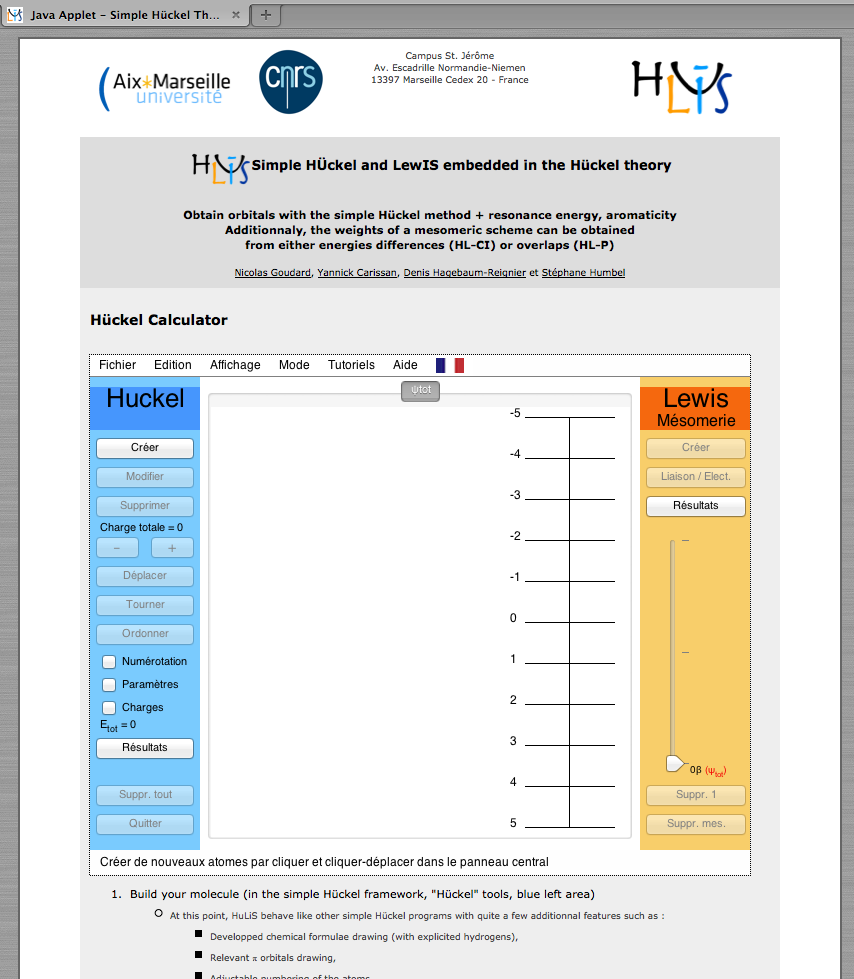Figure 1: HuLiS Web site : http://www.hulis.free.fr.

## The Hückel-Lewis approach

This scheme slightly modifies the Hückel theory : we need it to apply to Lewis structures rather than to atomic π orbitals. It is a simple scheme that computes the contributions (say the weights) of the contributing structures. It is detailed in the J. Chem. Educ. paper, but we shall remind here a few concepts.

### The scheme

First focus on the final objects :
OM-CLAO and Hückel: Φ=caχa + cbχb => Ψd (1)
Mesomerism: Ψm=cIΨI + cIIΨII (2)

The first equation expresses the Molecular Orbital as a linear combination of (local) atomic orbitals. From the molecular orbitals {Φ's} we can define a fundamental (delocalized) electronic configuration, Ψd. The second equation reads the resonating state as a linear combination of local contributing (resonant) structures. The similarity with the OM-CLAO is evident, and one shall just report to contributing structures what is normally done in Hückel with AO's.

Because contributing structures are electronic configurations, and because in quantum chemistry we call this type of approach a Configuration Interaction (CI), we named this scheme Hückel-Lewis Configuration Interaction (HL-CI).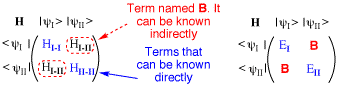Scheme 4 : The HL-CI matrices

We need to put in the HL-CI Hamiltonian Matrix i) the energies of each configuration (diagonal terms), and ii) their interacting terms (off-diagonal). By analogy to Hückel Theory, these terms are all negative. The overlap matrix of the scheme is set to the identity matrix, as it is the case in Hückel.

1. The energy of a configuration is read as the sum of the electron's energies when they are set in each localized orbitals (as lone pairs or π bonds). Those quantities are found from localized Hückel Hamiltonian matrix (setting βij to zero for atoms that are single bonded in the Lewis structure).
2. The interacting term is not known from scratch, but can be chosen in such a way that the mesomerism corresponds to the delocalized state, that is to say Ψd, Ed corresponds to Ψm, Em.
As detailed in a previous paper, it can be noted that once the Hamiltonian matrix is known, we can directly incorporate the energy Em to the secular equations, and get the coefficient CI and CII.

### Weights

The weight of a structure is the square of its coefficient in the wave function. This definition, consistent with the normalization of the state Ψm: CI2 + CII2=1, is only valid in the orthogonal basis (and corresponds to the standard orbital normalization in simple Hückel with an AO's basis set).

## The Hückel-Lewis Projected approach

In the following we present the scheme for a 2-structure mesomerism. It extends naturally to n-structure mesomerism, see 

### The scheme

Contrary to the HL-CI approach that uses energies, in the HLP approach we consider overlaps between multielectronic wave functions, written as Slater determinants of spin orbitals, delocalized (Φi), or localized (φi). Wave functions to use are :
• Ψd=|Φ1Φ2...Φn| (for the delocalized wave function)
• Ψm=cIΨI + cIIΨII (for the mesomerism wave function)
with ΨI=|φI1φI2...φIn|

We target the equality (never perfectly reached) |Ψd>=|Ψm>, and multiply it on the left by the bra of the resonating functions < ψI|, < ψII|, etc.
 The system of equations to solve is <ψI|Ψd>=<ψI|Ψm> <ψII|Ψd>=<ψII|Ψm>Hence, <ψI|Ψd>=<ψI|cIΨI + cIIΨII> <ψII|Ψd>=<ψII|cIΨI + cIIΨII> It comes, <ψI|Ψd>=cI<ψI|ΨI> + cII<ψI|ΨII> <ψII|Ψd>=cI<ψII|ΨI> + cII<ψII|ΨII>
Which gives cI, cII.

This system of equations can be set (and solved) if we know the overlaps <ψid> and <ψij>.
Because |Ψd>≠|Ψm> (a strict equality is not obtained), |Ψm> is not normalized. Hence cI, cII are that of the unnormalized |Ψm>.
One shall note that before normalization, <ψmm>=<ψmd>

### Overlap between determinants

Overlaps between 2 determinants are computed using the orthonormality of the atomic orbitals in the Hückel framework (<pi|pj>=δij).

Let's consider a 2-electron system in closed shell. The normalization of the determinants (1/N! ) gives a normalisation of 1/2

The overlap S= <1Φ1| |1φ1| >
S=1/2 [<Φ1(1)Φ1(2)− Φ1(1)Φ1(2)|φ1(1)φ1(2)−φ1(1)φ1(2)>]

We shall now remember that overlaps are one particule integrals, and consider the spin orthonormality (that is <α(i)|β(i)>=0).

It comes
S=1/2 [<Φ1(1)|φ1(1)><Φ1(2)|φ1(2)>+<Φ1(1)|φ1(1)><Φ1(2)|φ1(2)>]

Here, S= <Φ11>2

Example: Consider for the delocalized orbitals Φ1=0.54p1+0.84p2 (which represents the bonding orbital of formaldehyde if the atoms are numbered C= atom1 and O= atom 2),
and for the localized one φ1=p2 (which would be doubly occupied in the major ionic structure of formaldehyde ).
S= <Φ11>2 =<0.54p1+0.84p2|p2>2 =(0.54<p1|p2>+0.84<p2|p2>)2
S= 0.842

### Weights

Since we now consider the overlap between structures, the weight the Ith structure is computed following the Coulson-Chirgwin definition: wI=cIJ=1cJ SIJ, where the sum runs over all the mesomerism structures.

### Trust factor : τ

This is the overlap between the delocalized wave function and the localized one. τ=<Ψdm>
It gives the similarity between the two wave functions. If τ=1, the mesomerism wave function is exactly equal to the delocalized wave function. This situation is the almost never reached target.
The computation of τ is dedicated to HLP. However, when both HL-CI and HLP are selected, τ is additionally computed for the HL-CI wave function.

## Student's work in Organic Chemistry course

In this lab you will learn to use the HuLiS Java applet. By following these instructions you will get familiar with the applet and the HL-CI method. Along this document the following notation is used, is used for buttons and `Panel` for panels.

### A first look at the interface

The HuLiS applet is divided into three different panels : `Hückel`, `Graphical` and `HL-CI` (Figure 2).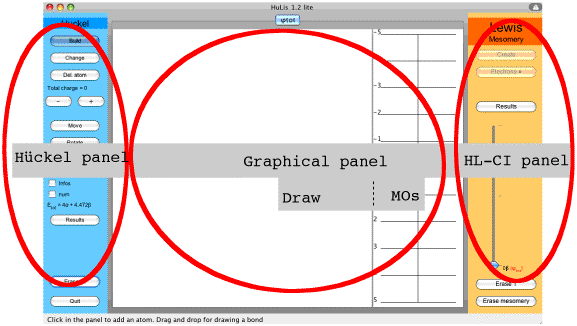Figure 2 : The HuLiS applet : the three panels.

• The `Hückel` panel. On the left of the applet, buttons are related to the molecule as considered with simple Hückel.
• : draw atoms and bonds on the left part of the ```graphical panel with the mouse ```
• ``` : modify the atom type (in the Hückel sense i.e. the value of α) modify the interaction between atoms (i.e. the value of β) ```
• ``` : delete an atom ```
• ```: increase/decrease the number of π electrons in the complete system ```
• ``` : move the whole molecule or just one atom ```
• ``` : rotate the whole molecule or just one atom ```
• ``` : complete reordering of the atoms of the molecule ```
• ``` : opens a window with Hückel calculation details ```
• ``` : erase everything, the applet is back to its initial state ```
• ``` : quit the applet ```
``` ```
• ```The HL-CI panel On the right of the applet, buttons are related with the HL-CI method. : add a Lewis structure in this new HL-CI description of the molecule : Add an electron on an atom (up to 2) modify the bond type (single/double) : opens a window with the HL-CI calculation details : erase the Lewis structure in the active tab : erase all the Lewis structures ```
• ``` The central Graphical panel It is divided into 2 parts. On the left part (Draw) it is possible to draw and modify the molecule or the active Lewis structure. On the right part (MOs) appears the molecular π orbitals spectrum. A click on an energy level in MOs draws the corresponding orbital in Draw. ```
``` Example : the acrolein molecule Standard Hückel calculations: As we have seen, the drawing of the molecule is done with the button. For the Hückel calculation, the tab used is entitled φtot. Click on the button and draw the butadiene molecule. To place an atom just click on the drawing panel, to place a bond between two atoms draw a line between them. Now the applet should look like Figure 3.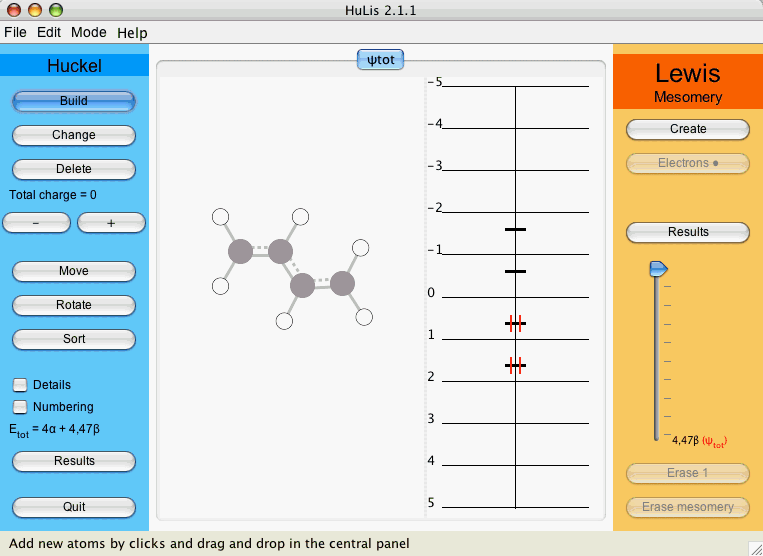Figure 3 : The applet after drawing butadiene. Up to now only carbon atoms were drawn. Since we aim at studying acrolein, we change the sp2 atom 4 (or 1) into a sp2 (one π electron) oxygen. To do so, click on and on the atom you want to change. A window appears, with all the possible atom types (Figure 4).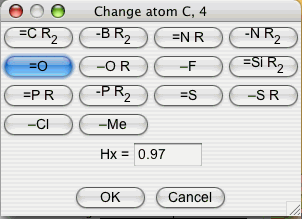Figure 4 : The atom types window Click on and on to get a monovalent sp2 Oxygen atom. After this step, the atom should have changed color indicating the new atom type. You might noticed that during the drawing of the molecule, as well as when changing the atom type, the molecular orbitals panel dynamically adapts to the changes. Indeed, the applet does a Hückel calculation after each action on the interface, thus any number given by the applet is always consistent with the molecule drawn. Let us look at the molecular orbitals of acrolein at the Hückel level. To do so, click on the energy level in MOs. The corresponding (delocalized) Molecular Orbital (MO) appears for each selected energy level.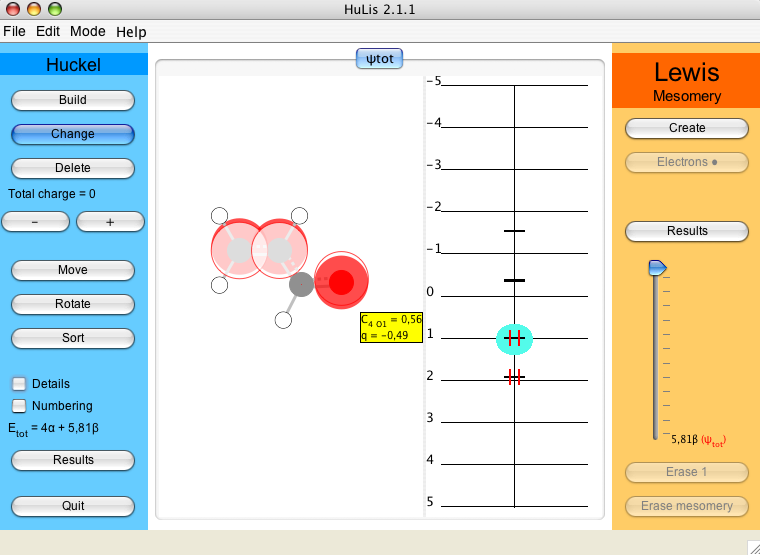Figure 5 : The second occupied molecular orbitals of acrolein. Since calculations are done with Hückel theory, molecular orbitals are delocalized over the whole molecule. MO Coefficients appear (yellow box) when the mouse stays over the orbital. Hückel CI calculations: Now, we want to express the Hückel electronic structure of acrolein into Lewis contributing structures. HuLiS can handle as many contributing structures as the user would possibly imagine. However, chemically interesting results are obtained with a small number of structure. Two-structure calculation: To enter in the Hückel Lewis CI part of the work, the HL-CI buttons will be used. In order to add a new Lewis structure, click on . A new tab is created with the skeleton, without any π electrons. This tab is entitled ψ1 and is active.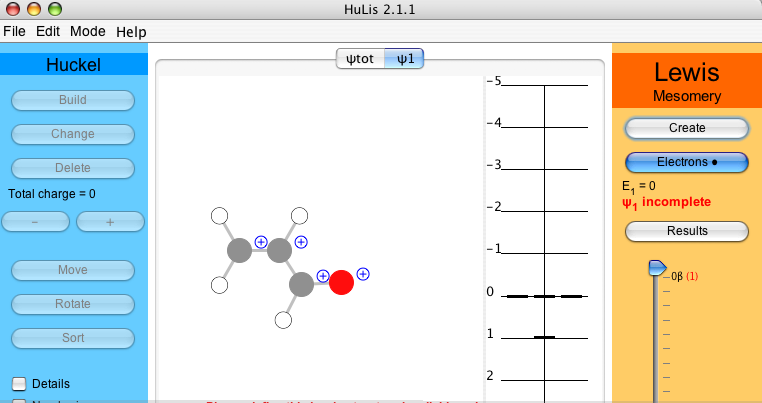For acrolein, four π electrons must be placed either on atoms or as bonds. Click on and make the covalent structure by selecting the first and the last bonds. They change into double bonds. In order to do any meaningful calculation, one must have at least two Lewis structures. Proceed as previously to create the ionic structure, tab ψ2. The second most relevant structure for acrolein has the middle bond as a double bond and an electron pair on the oxygen. As before, click on and select the middle bond, which becomes double, and click twice on the oxygen to put two electrons on it. The atomic charges are indicated. When the fourth electron is added, the weight of the active structure is displayed beneath . In the two-structure case of acrolein one gets 78.7% for ψ1 and 21.3% for ψ2 (Figure 6). Thus, in a two-structure description, the Hückel solution can be seen as a normalized linear combinaison such as ψHückel = 0.89 ψ1 + 0.46 ψ2 (see of the Lewis part).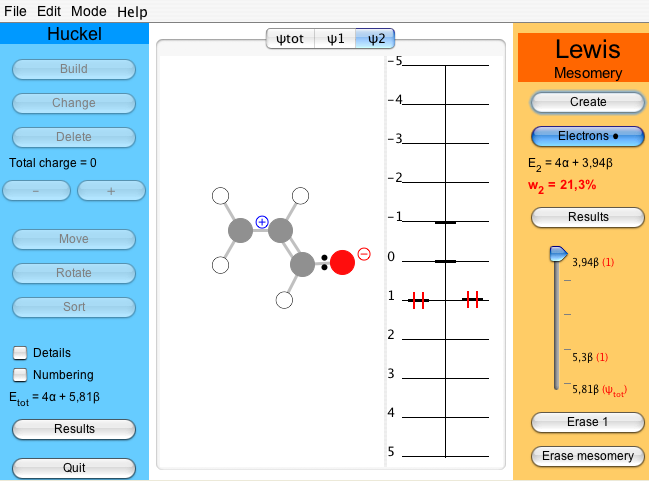Figure 6 : A two-structure study of acrolein : w1=78.7% vs w2=21.3% Three-structure calculation: To go further and play with the description of acrolein, we can include a third ionic Lewis structure in which the end-carbon carries the negative charge. This structure does not account for electronegativity and shall have a small contribution. To check so, click : the ψ3 tab appears. Draw the appropriate bond and lone pair. We get the weights of each structure : 77.5/17/5.5. The smallest value is obtained for the last structure (5.5%), which is consistent with the resonance rules. An ab initio NRT calculation give a similar result : 84/11/5 at the B3LYP/6-31G(d) level.[2,3,4] ```
``` ```
``` Conclusion Through the HL-CI chapter, the concepts of mesomerism can be putted into equations and related to the Hückel lesson. The approach resituates the mesomerism in the theoretical chemistry field. We are currently defining pertinent classroom exercices that can be added in the Hückel lesson. These exercices will be proposed on the web site in due time. The java code can be used to illustrate the rules that define what is a major structure, what is a minor one. In the lab example we have seen how to use the HuLiS applet to decompose the Hückel description of the π system of acrolein. This simple scheme creates an additional link between theory and usual organic chemistry concepts that are Lewis structures, resonance and even aromaticity. The current version of HuLiS introduces a trust parameter (stabilized in Dec. 2014). This parameter indicates how much of the delocalized state is incorporated in a given resonance pattern. Aromaticity is of a special interest here : the two kekule structures of benzene can be shown to poorly describe the delocalized structure of benzene (the trust parameter is as small as 60% - see table 1 below). Adding the Dewar structures (3 covalents and 6 ionics) increases the trust parameter to 72%. A better value (94%) is reached with as many as 35 structures. This large number is to be attributed to the aromatic nature of benzene - this is not a simple mesomerism. The application of the trust parameter in the butadiene resonance is shown in Table 2. A single structure is already "trustable" by about 80%. The Hückel interface is versatile so virtually any atom can be parameterized at will. Nitrile groups for instance can be parameterized following Heilbronner et al. : αN= α+β βN=1.2β βC-CN=β/√2 Table 1: Benzene resonance.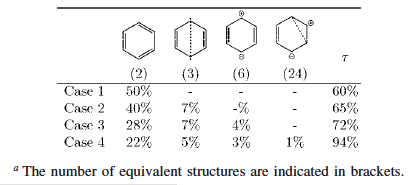Table 2: Butadiene resonance.```

# References

 Humbel, S. J. Chem. Educ. 2007, 84, 1056-1061.[ Getting the Weights of Lewis Structures out of Hückel Theory. Hückel-Lewis Configuration Interaction (HL-CI) ] don't forget the correction due to printing mistake in the table.
 Hagebaum-Reignier, D.; Girardi, R.; Carissan, Y.; Humbel, S. J. Mol. Struct. (THEOCHEM) 2007, 817, 99-109.[ Hückel Theory for Lewis Structures: Hückel-Lewis Configuration Interaction (HL-CI) ]
 Glendening, E.D. ; Weinhold, F. J. Comp. Chem. 1998, 19, 593-609. See also the two papers after this one.
 The predominance of the octet rule will be valid all over this paper that deals only with situations where the octet rule is obeyed. For specific comments on the subject see for instance (a) Suidan, L.; Badenhoop, J.K.; Glendening, E.D.; Weinhold, F. J. Chem. Educ. 1995, 72, 583-586; (b) Weinhold, F. J. Chem. Educ. 1999, 76, 1141-1146; (c) (d) Weinhold, F. J. Chem. Educ. 2005, 82, 526-527 and the reply from Purser, G.H. J. Chem. Educ. 2005, 82, 528-529; (e) Purser, G.H. J. Chem. Educ. 1999, 76, 1013-1018. (f) Purser, G.H. J. Chem. Educ. 2001, 78, 981-983.
 Carissan, Y. ; Hagebaum-Reignier, D. ; Goudard, N.; Humbel, S. J. Chem. Phys. A 2008, 118, 13256–13262. [Hückel-Lewis-Projection Method: A "Weights Watcher" for Resonant Structures ]
 Heilbronner, E.; Yang, Z. Z. Angew. Chem. Int. Ed. Engl. 1987, 26, 360-362. [The Influence of Substituents on Double-Bond Localization, e.g. in s-Indacene]